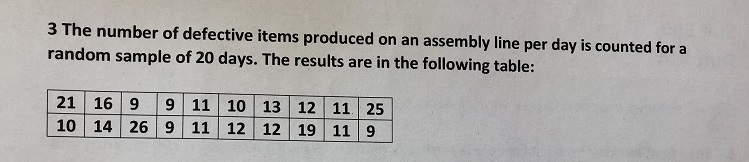# 3 The number of defective items produced on an assembly line per day is counted forarandom sample of 20 days. The results are in the following table:21 16 99 11 10 13 12 11 2510 14 26 9 11 12 12 19 11 9

Question
1 views

d) Based on the Boxplot, is it reasonable to treat this sample as if it comes from an approx. normal population? Briefly explain.

5 Number Summary of Boxplot:

Min: 9

Q1: 10

Med/Q2: 11.5

Q3: 15

Max: 26help_outlineImage Transcriptionclose3 The number of defective items produced on an assembly line per day is counted fora random sample of 20 days. The results are in the following table: 21 16 99 11 10 13 12 11 25 10 14 26 9 11 12 12 19 11 9 fullscreen
check_circle

Step 1

From the given five number summary, the boxplot is,

Step 2

The rule for determining the skewness from the Boxplot:

• The data is right skewed if the median is closer to the 1st quartile than the 3rd quartile or the upper whisker is longer than the lower whisker.
• The data is left skewed if the median is closer to the 3rd quartile than the 1st quartile or the lower whisker is longer than the upper whisker.
• The data is approximately symmetric if the median is the middle point of 1st quartile and the 3rd quartile or the length of the upper whisker and the lower whisker is approximately same.
Step 3

From the above boxplot, it can be said that the median is closer to the 1st quartile than the 3rd quartile and the upper whisker is longer than the lower whisker. There are two outliers in the data.

Therefore, the d...

### Want to see the full answer?

See Solution

#### Want to see this answer and more?

Solutions are written by subject experts who are available 24/7. Questions are typically answered within 1 hour.*

See Solution
*Response times may vary by subject and question.
Tagged in

### Other